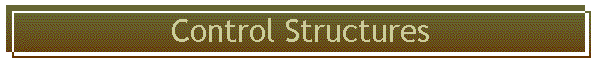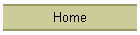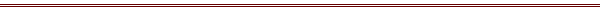A summary of the control structures available## no logical variables

Unlike Fortran, C goes not have logical variables.  Instead, zero is false and any non-zero value is  true.  A logical statement will set a true result to unity.   In the expression i = ( a<= 0.) i will be set to 0 if a is positive, and to 1 if less than or equal to zero.

The variables TRUE and FALSE are defined in the header

## for loop

/* loop from 1 to 10 with declaration, notice declared 11 since -10 is 11 */
double a;
for( i=1; i<=10; i++ )
{
a[i] = 0;
}

/* more usual way, from 0 to less than 10 */
double a;
for( i=0; i<10; i++ )
{
a[i] = 0.;
}

loops usually begin at 0 and test for being less that upper limit - then upper limit is same as dimension of variable

## while statement

This loop will continue cycling until the condition is not satisfied:

while( a<= 0. )
{
code goes here
}

## do statement

This statement will cycle at least once, since the test occurs at the end

do
{
code goes here
} while ( logical test)

## break and continue

The continue command causes the code between it and the end of the loop to be skipped, and the loop started on its next iteration.  It is the same as the cycle command in Fortran 90.

for( i=0; i<=6; i++ )
{
code here always executed in loop . .
if( a <= 0 ) continue;
code here only executed if a positive, but loop continues
}

The break command causes the current loop to be exited.   It will go up to the next higher loop if one is present. It is the same as Fortran 90's exit command.  The following combines a while loop that cannot exit (since it tests on true) with a break command to provide the only way out:

while (TRUE )
{
code goes here
if( a<=0. ) break;
}

## if else statement

/* the following is a simple if statement*/
if( a<= 0. )
{
code for true condition goes here
}

/* this is an it - else pair */
if( a <= 0. )
{
code for true branch goes here
}
else
{
code for false branch goes here
}

## goto

It is very rare for a well written code to need to use this statement, but it does need to be used once every few years.  It is needed mostly when one needs to jump our of several loops.  First, the token is "goto" so it cannot be written as "go to" as in Fortran.  The syntax is

goto label;

where label is anything that could have been a variable, but ends with a colon as in

label:

## conditional operator ?

A conventional if - else clause looks like the following, which sets x  to the smaller of y or z:

if( y < y )
{
x = y;
}
else
{
x = z;
}

This can be collapsed into the following C

x = (y < z ) ? y : z ;

The full syntax is
l(logical expression) ? {expression one} : {expression two}

The conditional operator results in code that will astonish a seasoned FORTRAN programmer.

## The comma operator

This allows you to put several statements where one would be expected.  It would be written as

a = 0. , b = 9. , c = 1. ;